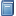Buchempfehlung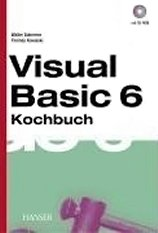Visual Basic 6 Kochbuch
Viele praktische Tipps zum Programmieren mit Visual Basic 6, die sich oft auch auf FB übertragen lassen. [Mehr Infos...]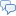FreeBASIC-Chat
Es sind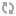Benutzer im FreeBASIC-Chat online.
(Stand:  )

# fb:porticula NoPaste

 Uploader: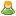Volta Datum/Zeit: 30.09.2010 20:39:49
```'PROGRAM test_quad '     Three tests of the basic quadruple precision module. '     1.       (A + B)^2          = (A - B)^2 + 4.A.B '     2.  (A^2 - B^2) / (A - B)   = A + B '     3.  SQRT(A^2 + 2.A.B + B^2) = A + B #Include "quad_1x.bi" Using quad_precision Screen 0 Width 90,40 Color 15,1 Cls '' This captured from fortran code: #define EPSILON_HALF 2.22044604925031308E-016 Print EPSILON_HALF '' This derived by trial and error: Print 2^-52 Print Randomize 4711 '' I used this to verify that my code was producing random quads '' in the same general range as the fortran test code. It does, '' but I still don't understand why this range was chosen. Dim As quad r, mn, mx mn = 2000000000.0 For i As Integer = 1 To 1000000   r.hi = Rnd   r.hi = (r.hi-0.5) / r.hi   r.lo = r.hi * EPSILON_HALF   If r < mn Then mn = r   If r > mx Then mx = r Next Print "min = ";mn Print "max = ";mx Print Dim As quad a, b, lhs, rhs, diff For i As Integer = 1 To 10   a.hi = Rnd   a.hi = (a.hi-0.5) / a.hi   a.lo = a.hi * EPSILON_HALF   b.hi = Rnd   b.hi = (b.hi-0.5) / b.hi   b.lo = b.hi * EPSILON_HALF   '' (A + B)^2 = (A - B)^2 + 4.A.B   lhs = (a + b) * (a + b)   rhs = a * b   rhs = 4.0 * rhs   rhs = (a - b) * (a - b) + rhs   diff = lhs - rhs   Print "lhs = ";lhs,"diff = ";diff   '' (A^2 - B^2) / (A - B) = (A + B)   lhs = (a*a - b*b) / (a - b)   rhs = a + b   diff = lhs - rhs   Print "lhs = ";lhs,"diff = ";diff   '' SQRT(A^2 + 2.A.B + B^2) = A + B   lhs = a * b   lhs = 2.0 * lhs   lhs = Sqr(a*a + lhs + b*b)   If rhs.hi < 0 Then     rhs = -rhs   End If   diff = lhs - rhs   Print "lhs = ";lhs,"diff = ";diff Next Sleep```